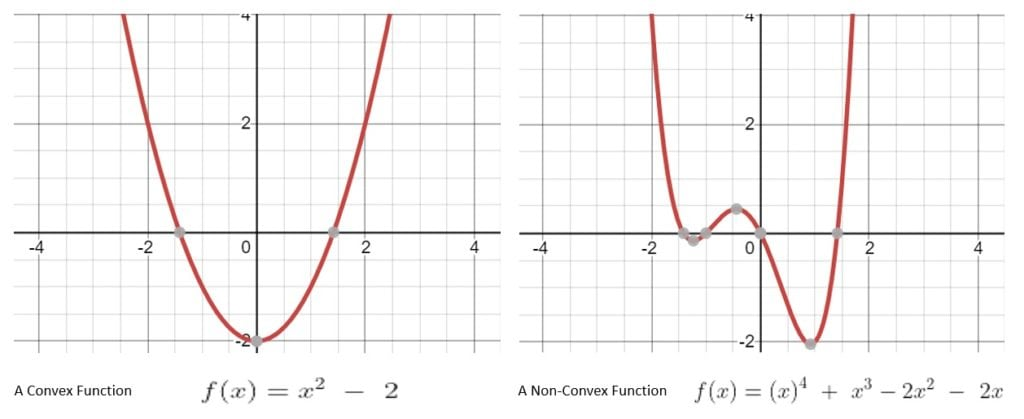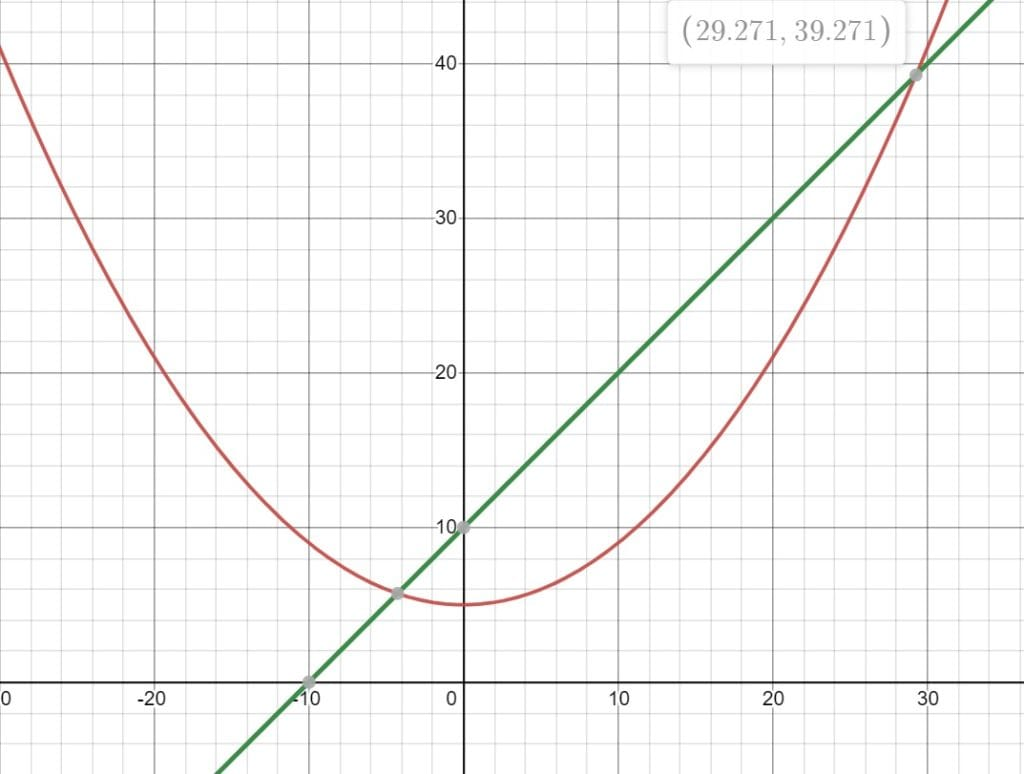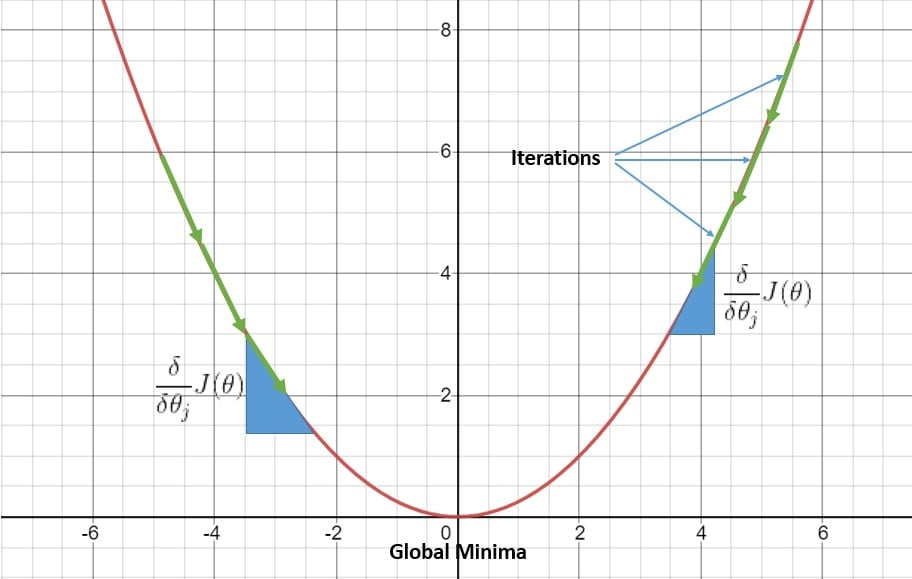## 1. Introduction

In this tutorial, we’ll try to build a general intuition behind the gradient descent algorithm. By understanding the mathematics behind gradient descent, we’ll understand why it works for problems like linear regression.

More importantly, we’ll see why it’s effective compared to other ways to solve such problems.

## 2. Optimization

Optimization is the general process of identifying the combination of inputs to achieve the best possible output under certain constraints and conditions. Several practical problems require an optimization technique to solve, like the traveling salesman problem for instance.

The objective here is to find the shortest and most efficient route for a person to take given a list of destinations.

Perhaps an easier way to understand optimization is using a simple mathematical formulation like a linear function:This function is known as the objective function in linear programming. The idea is that it defines some quantity that needs to be maximized or minimized under the given constraints. There are other classes of optimization problems that involve higher-order functions, like quadratic programming.

There are several ways to solve optimization problems, like linear programming or quadratic programming; however, it’s imperative to understand that an important feature of these functions is that they are convex functions.

A convex function is one where if we draw a line from, to, then the graph of the convex function lies below that line. A function that doesn’t exhibit this property is known as a non-convex function:It’s fairly intuitive to understand why this is important in optimization problems. A convex function can have only one optimal solution that is globally optimal. This makes solving such a problem much easier comparatively.

On the other hand, a non-convex function may have multiple locally optimal solutions. This makes it incredibly difficult to find the globally optimal solution.

## 3. Linear Regression

Linear regression is a statistical technique to fit a linear equation to the observed set of data. The idea is to define a linear relationship between a scalar response and one or more explanatory variables. For instance, we may try to model the relationship between rain and explanatory variables like temperature, humidity, and altitude.

Linear regression is quite elegant because it takes quite a simple form mathematically:Hereis the scalar response, andis the explanatory variable. The slope of the lineand the interceptare model parameters that we intend to learn from the observed set of data.

So how do we solve the problem of linear regression? We need to transform this into an optimization problem. One of the most widely used formulations for linear regression is the method of least-squares. Basically, we try to minimize the cost function of the linear model:Here we try to minimize the total error between our estimate and the real value across all the input data. The objective is to find the set of model parametersthat gives us the least value for the cost function.

## 4. Solving the Cost Function

The cost function in the linear least-squares method is a quadratic function. Thus, the problem of optimizing this cost function is a quadratic programming problem. There are several ways to solve this problem. We can begin by employing some of the simpler options like analytical, graphical, or even numerical.

Let’s see how we can solve a quadratic programming problem analytically. We’ll begin by taking a simple quadratic objective function along with a linear constraint:We’re using an objective function with a single decision variable to keep things simple; however, in practical applications, the number of decision variables is much higher. Following the analytical approach, we’ll rearrange the objective function and the constraints to give us a quadratic equation:Solving this quadratic equation is fairly simple. We can also visualize this graphically to understand the solution:However, imagine if we had hundreds of decision variables instead of one. That would mean we’d no longer be able to solve this problem efficiently without using numerical software. Even with numerical software, it may take an exponentially higher amount of time to solve such a problem with every additional decision variable.

Of course, there are several other ways to solve a quadratic programming problem, like Interior Point, Active Set, Augmented Lagrangian, Conjugate Gradient, and an extension of the Simplex Algorithm. We won’t go into the details of these here.

One of the iterative approaches to solving such a problem is gradient descent.

Gradient descent is an iterative algorithm to find the function’s coefficients that minimize the corresponding cost function. These coefficients are nothing but the parameters of the linear model that we need to learn. We start with all the coefficients assuming some small random values. This helps us to calculate the value of the cost function with these coefficients.

The next step is to adjust these coefficients so that we can lower the value of the cost function. For this, we need to know in which direction we should adjust the coefficient values. Here derivatives of the cost function with respect to coefficients helps us:Basically, the partial derivative is a tool from calculus that gives us the slope of the cost function at a given point concerning a coefficient. We update the coefficients using the derivative and a learning rate parameter to control the rate of change.

We repeat this process until we reach the optimal values for our coefficients. This may mean that the value of the cost function becomes zero, or more practically, close to zero. This gives us a straightforward, but powerful, optimization technique to solve linear regression problems with several decision variables. Of course, we must have a differentiable cost function.

## 6. Why Gradient Descent Works?

Now we’ve explored linear regression, its cost function, and how to solve it using the gradient descent algorithm. The question remains why does the gradient descent algorithm work for the linear least-squares cost function? Actually, the intuition behind this is not very complicated to understand. We have seen how a convex function can guarantee us a single globally optimal solution.

Basically, the cost function in the case of the least-squares method turns out to be a convex function. This assures us that we’ll only have a single optimal solution.

While the analytical approach becomes impractical as the problem space grows, the iterative approach of gradient descent works quite well. While updating the coefficients in the right direction, we’re sure to reach the global minima:The only caveat is to choose the right learning rate parameter. With a large learning rate, we risk missing the optimal solution due to large strides, while with a small learning rate, we may take much longer to reach the optimal solution.

Of course, the gradient descent can also work for non-convex cost functions; however, this may result in finding a locally optimal solution instead of the globally optimal solution. This makes working with the gradient descent algorithm in a non-convex model quite challenging.

## 7. Conclusion

In this article, we examined the basics of optimization problems and convex analysis. We also discussed the problem of linear regression and how to solve its cost function.

Finally, we analyzed why the gradient descent algorithm works well for solving such problems compared to the analytical approach. This helps us to build the intuition behind the gradient descent algorithm.